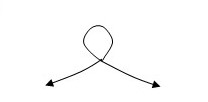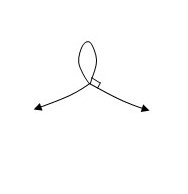# If A is a positive number, the parametric curve (x(t),y(t)) (t^3 - t, \frac{A}{1 + t^2}) looks...

## Question:

If A is a positive number, the parametric curve (x(t),y(t)) = {eq}(t^3 - t, \frac{A}{1 + t^2}) {/eq} looks like:For one value of A, the "self-intersection" of the curve is perpendicular. The picture looks like:Find that value of A.

## Parametric Curves

A curve can be self-intersecting in a point by tracing the curve with two different parameters.

To obtain the point of self-intersection of a parametric curve,

we will solve the equation of the coordinate points to be equal in Cartesian coordinates, but for two different parameters.

A self-intersecting curve will have different tangent lines to the curve at the point of intersection.

(a) To find A such that t that the curve {eq}\displaystyle x=t^3-t, y=\frac{A}{1+t^2}, {/eq} self-intersects in a point where the slopes are perpendicular,

we will obtain the point of self-intersection ,first.

For this, we need to find two parameters, {eq}\displaystyle s,t, s\neq t, {/eq} such that

{eq}\displaystyle \begin{align*} &\displaystyle \begin{cases} t^3-t=s^3-s \\ \frac{A}{1+t^2}=\frac{A}{1+s^2} \\ \end{cases}\\\\ & \implies \displaystyle \begin{cases} t^3-t=s^3-s \\ t^2=s^2\iff t=\pm s, \text{ which substituted in the first equation, } \\ \end{cases}\\\\ & \implies \displaystyle \begin{cases} \pm s^3\mp s=s^3-s \implies s^3-s=s^3-s \text{ or }-s^3+s=s^3-s \iff s(s^2-1)=0\implies s^2=1, \text{ because } s=0 \text{ implies }t=0 \text{ whihc is not allowed} \\ t=\pm s \\ \end{cases}\\ \end{align*} {/eq}

So, the two parameters where the curve intersect itself are {eq}\displaystyle t=-1, t=1. {/eq}

To find A such that the tangent lines are perpendicular, we will determine the slopes.

{eq}\displaystyle x'(t) =\frac{d}{dt}(t^3-t)=3t^2-1\\ \displaystyle y'(t) =\frac{d}{dt}\left(\frac{A}{1+t^2}\right)=-\frac{2At}{(t^2+1)^2}\\ \text{ so, the slope is } \displaystyle \dfrac{y'(t)}{x'(t)}= -\frac{2At }{ (t^2+1)^2(3t^2-1)} \implies\\ \displaystyle \dfrac{y'(-1)}{x'(-1)} =\frac{2A}{4\cdot 2}=\frac{A}{4}\\ \text{ and }\displaystyle \dfrac{y'(1)}{x'(1)} =-\frac{2A}{4\cdot 2}=-\frac{A}{4}\\ {/eq}

So, the two slopes of the tangents lines should have the product equal -1 for orthogonality

{eq}\displaystyle -\frac{A}{4}\cdot \frac{A}{4} =-1\implies A^2=16 \implies \boxed{A=-4 \text{ or } A=4}. {/eq}